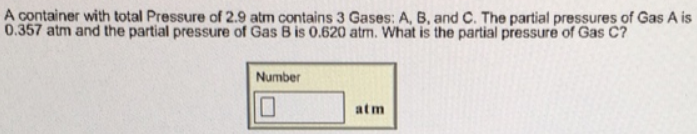# Problem: A container with total Pressure of 2.9 atm contains 3 Gases: A, B, and C. The partial pressures of Gas A is 0.357 atm and the partial pressure of Gas B is 0.620 atm. What is the partial pressure of Gas C?

###### FREE Expert Solution###### Problem Details

A container with total Pressure of 2.9 atm contains 3 Gases: A, B, and C. The partial pressures of Gas A is 0.357 atm and the partial pressure of Gas B is 0.620 atm. What is the partial pressure of Gas C?